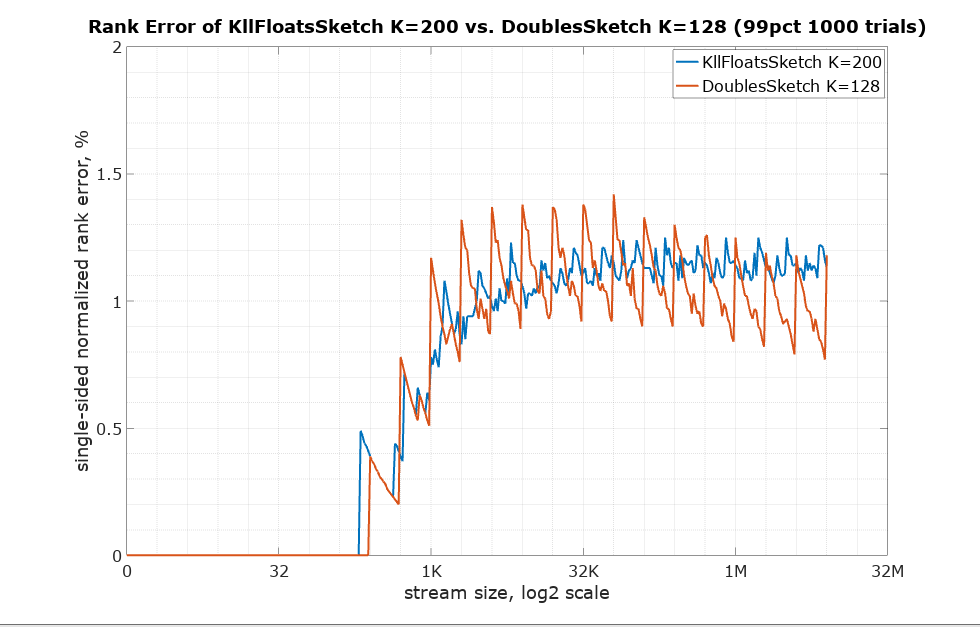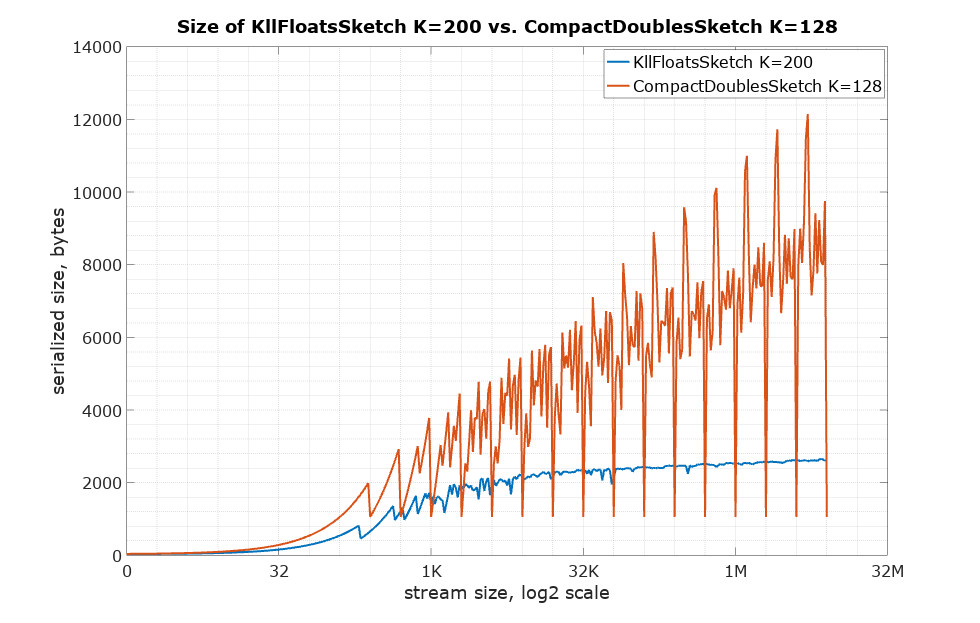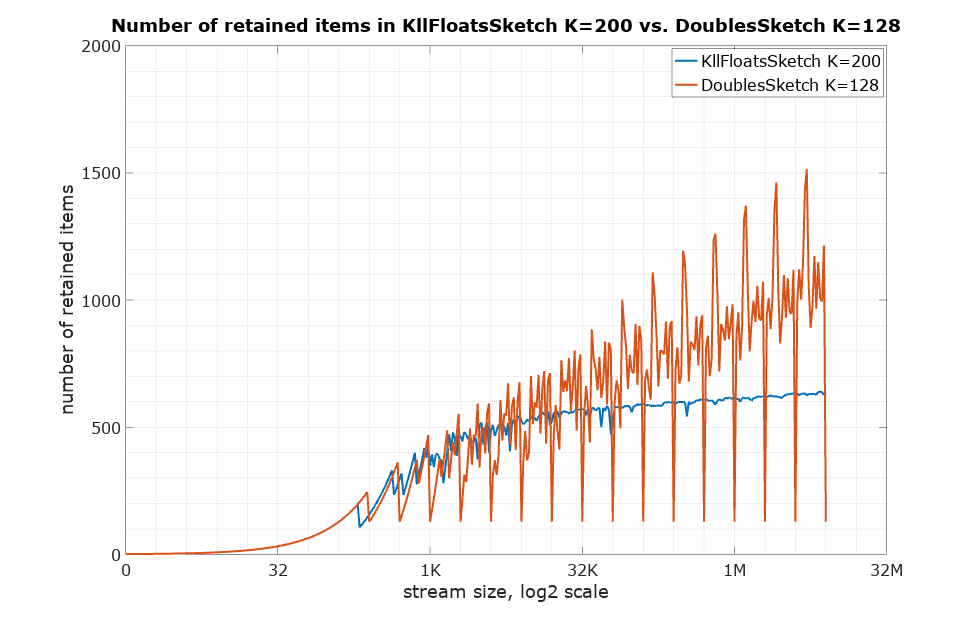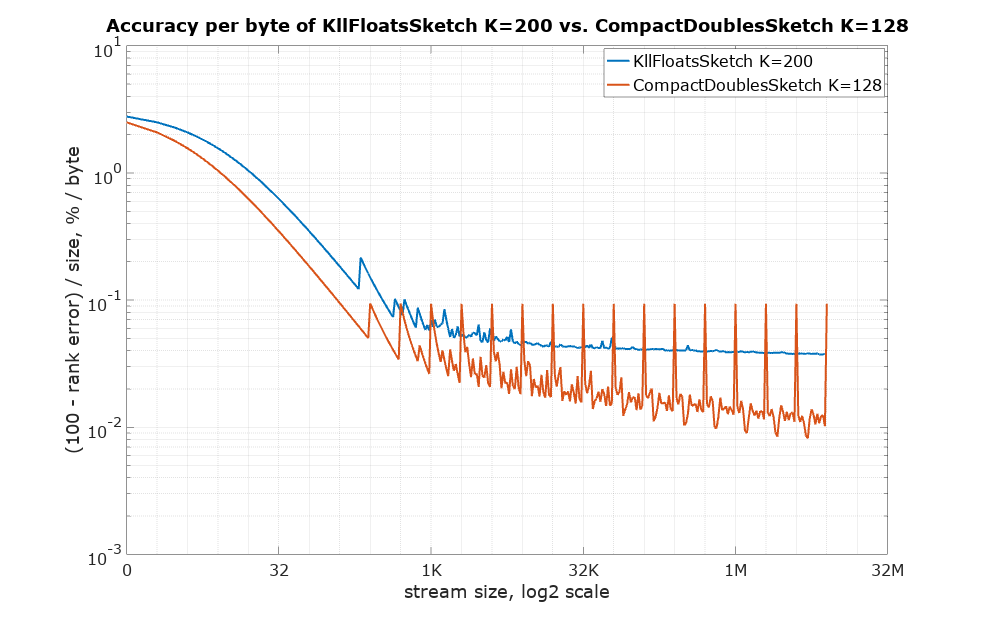## KLL sketch

Implementation of a very compact quantiles sketch with lazy compaction scheme and nearly optimal accuracy per bit. See this paper. The name KLL is composed of the initial letters of the last names of the authors.

The usage of KllFloatsSketch is very similar to DoublesSketch. Because the key feature of this sketch is compactness, it was implemented with float values instead of double values. The parameter K that affects the accuracy and the size of the sketch is not restricted to powers of 2. The default of 200 was chosen to yield approximately the same normalized rank error (1.65%) as the default DoublesSketch (K=128, error 1.73%).

### Java example

import org.apache.datasketches.kll.KllFloatsSketch;

KllFloatsSketch sketch = new KllFloatsSketch();
int n = 1000000;
for (int i = 0; i < n; i++) {
sketch.update(i);
}
float median = sketch.getQuantile(0.5);
double rankOf1000 = sketch.getRank(1000);


### Differences of KllFloatsSketch from DoublesSketch

• KLL has a smaller size for the same accuracy
• KLL is slightly faster to update
• The KLL parameter K doesn’t have to be power of 2
• KLL operates with float values instead of double values
• KLL uses a merge method rather than a union object
• KLL does not offer direct, off-heap implementation
• KLL does not have separate updatable and compact forms

The starting point for the comparison is setting K in such a way that rank error would be approximately the same. As pointed out above, the default K for both sketches should achieve this. Here is the comparison of the single-sided normalized rank error (getRank() method) for the default K:DoublesSketch has two forms with different serialized sizes: UpdateDoublesSketch and CompactDoublesSketch. KllFloatsSketch has no such distinction. It is always serialized in a compact form, and it is not much bigger than that in memory. Here is the comparison of serialized sizes:Some part of the size difference above is due to using items of float type as opposed to double type. Here is the comparison of the number of retained items to see the difference with no influence of the size of the item type:Below is the accuracy per byte measure (the higher the better). Suppose rank error is 1%, so the corresponding accuracy would be 99%. Divide that by size in bytes to arrive at this measure of how expensive each percentage point is in terms of space needed:Below is the update() method speed: